# FAQ: In the congestion control model, what does it mean to evaluate r i at time t - tau i?

Jump to: navigation, search

(Contributed by Richard Murray, 2 July 2009)

Q: On page 79, line 14, the text says "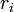is evaluated at time, representing the time required to receive additional acknowledgements. What does this mean?

A: The symbolrepresents the rate at which packets are cleared from the senders queue and it is equal to the window size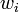divided by the round trip time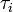. The window size is increased or decreased based on whether a packet arrived at the sender and the corresponding acknowledgement packet arrived back at the original sender, as described in equation (3.16):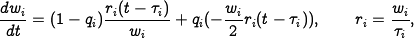The total time from when the packet was sent until the acknowledgement packet is received is the round trip time. Thus, the rate at which acknowledgements are received is the rate at which were sentms ago. This is the meaning of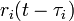.

Another point of clarification is that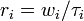(from equation (3.16)) and so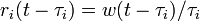(is a constant).